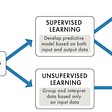# Introduction

We are aware power of PCA and wonders that were achieved by it domains of image compression, data analysis and supervised machine learning. PCA explains correlated multivariate data in fewer number of linearly uncorrelated variables which are linear combination of original variable. In simple terms it removes redundant information with catching most important features first i.e. the ones with highest variance. Here, are interesting examples by Victor to get to started with on importance of PCA, you can follow along with his series for mathematics behind PCA. First see the two images, answer the question and then look at explanation.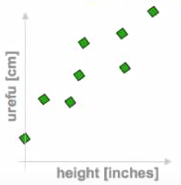For some population two properties are measured.What is the dimension of data ?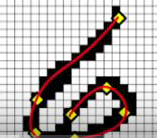Let’s say 20x20 image digit dataset, Leading to 400 grid boxes. What is the dimension of data ?

Explanation: Let’s say for first example we came know that urefu means height in Swahili. Clearly there is no need for two separate dimensions, one feature will do. Also, for second case with an image containing 400 dimensions. For bitmap images of 2⁴⁰⁰ possibilities only few are the ones which captures resembles to digits. Clearly, the dimensions in this case has to be less than 400 limited only to a few to capture all information about digits. Like, only specific curves, lines of a digit are present or not.

PCA with its statistical linear transformations based on variance and co-variance calculations has been very successful in detecting such redundancies. From above examples we can clearly see a need for generalization mechanism for information to be expressed in the form of features in a dataset. Let’s see how successful PCA was in such generalizations which leads to most important features being captured first and is there any need for better mechanisms.

# Power of PCA Explained

Let’s say you have a dataset involving a huge number of features to work with. It is mostly the case with image, video or audio data. You can’t apply our regular machine learning models directly onto it, you will definitely look for some meaningful preprocessioning step which will reduce your training time but still not reduce representation power of data i.e. accuracy/error trade-off must be less.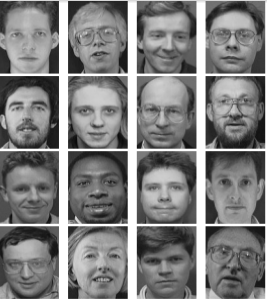100 x 100 images [10K Dimensions]. Data will have m x 10k dimensional matrix

The special thing about data related to images is that each pixel is related to its neighboring pixels, we should leverage this fact. Generally the case with image, audio and video datasets. This relation forces us to conclude that higher dimensions don’t have much information stored in them and we might not need them. If some dimensions are sparse we can discard them without losing out much information. Now the question is which dimensions to pick? Enter PCA. It picks up dimensions such that the data exhibits a high variance across these dimensions, and thereby more representation power is captured. It ensures that the covariance between the new dimensions is minimized. Which will ensure that the data can be represented using less number of dimensions.

The above result is incredible. Without any significant loss, the image gets reconstructed with 0.16% of the original number of dimensions. The above image is reconstructed with linear combination of eigen vectors which forms the basis. Imagine the amount of space and time we can save in storing and training our models, respectively, using this reduced form of data. But how does it all relate to autoencoders?

# How autoencoders are linked to PCA ?

Well, an autoencoder is a special type of feed forward neural network which encodes input x into hidden layer h and then decodes it back from its hidden representation. The model is trained to minimize the loss b/w the input and output layer.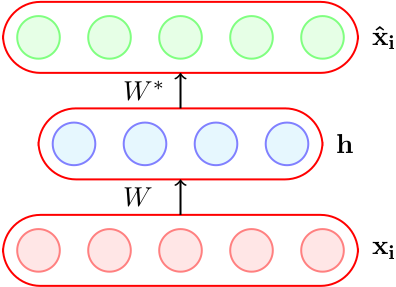An autoencoder where dim(h) < dim(xi) is called an under complete autoencoder

Now, let’s say with the help of hidden layer h, you are able to reconstruct xhat perfectly, h is lossless encoding of xi. It captures all important characteristics of xi. The analogy with PCA is clear, h behaves like PCA’s reduced dimensions matrix from which the output is reconstructed with some loss in value. Hence, the encoder part shows resemblance to PCA.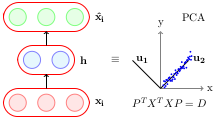How to achieve this equivalence? Can this equivalence be of any use?

# Which conditions make autoencoder a PCA?

Encoder part will be equivalent to PCA if linear encoder, linear decoder, square error loss function with normalized inputs are used. Which means PCA is restricted to linear maps only whereas autoencoders are not.

Due to these linearity constraints, we moved to encoders with sigmoid-like non-linear functions which give more accuracy in the reconstruction of data. See an illustration related to it.

# A Comparative Illustration

Here are comparative codes of PCA and an autoencoder for training MNIST dataset with mean square error as loss function. Following architecture 784→512→128→2→128→512→784 for autoencoders is being used in this illustration. In Python 3.x you need to install TensorFlow and Keras for this code illustration.

Setup

`import numpy as npimport kerasfrom keras.datasets import mnistfrom keras.models import Sequential, Modelfrom keras.layers import Densefrom keras.optimizers import Adam(x_train, y_train), (x_test, y_test) = mnist.load_data()x_train = x_train.reshape(60000, 784) / 255x_test = x_test.reshape(10000, 784) / 255`

PCA

`mu = x_train.mean(axis=0)U,s,V = np.linalg.svd(x_train - mu, full_matrices=False)Zpca = np.dot(x_train - mu, V.transpose())Rpca = np.dot(Zpca[:,:2], V[:2,:]) + mu    # reconstructionerr = np.sum((x_train-Rpca)**2)/Rpca.shape/Rpca.shapeprint('PCA reconstruction error with 2 PCs: ' + str(round(err,3)));`

Autoencoder

`m = Sequential()m.add(Dense(512,  activation='elu', input_shape=(784,)))m.add(Dense(128,  activation='elu'))m.add(Dense(2,    activation='linear', name="bottleneck"))m.add(Dense(128,  activation='elu'))m.add(Dense(512,  activation='elu'))m.add(Dense(784,  activation='sigmoid'))m.compile(loss='mean_squared_error', optimizer = Adam())history = m.fit(x_train, x_train, batch_size=128, epochs=5, verbose=1, validation_data=(x_test, x_test))encoder = Model(m.input, m.get_layer('bottleneck').output)Zenc = encoder.predict(x_train)  # bottleneck representationRenc = m.predict(x_train)        # reconstruction`

Change all the activation functions to `activation='linear'` and observe how the loss converges precisely to the PCA loss. That is because linear autoencoder is equivalent to PCA as explained above.

# Conclusion

Above theory and example gives an idea why there was a need to move from PCA to autoencoders. Presenting the case for non-linear functions as compared to linear transformations as in PCA. Vanilla encoders no doubts are primitive in terms of its functioning even on MNIST like datasets where sparse or denoising autoencoders will simply overpower in case of over complete autoencoders for example. But, still the foundation of non-linear activation functions paves the way deep learning models to outperform other convention ML models. Thanks!!

# Acknowledgements

I have merely scratched the surface & tried to explain the basics of this concept, for deep dive into theory refer CS7015(DL, IITM) and for details of implementation refer following thread by amoeba.

--

--

--

## More from Analytics Vidhya

Analytics Vidhya is a community of Analytics and Data Science professionals. We are building the next-gen data science ecosystem https://www.analyticsvidhya.com

## Recommended from Medium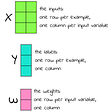## Atlas — Neural Network Reconstructing a 3D Scene From Image 📸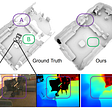## Increase HCP Targeting Performance With Machine Learning## What is Machine Learning?## Introduction to Decision Trees in Machine Learning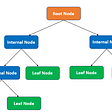## Building Spam Classifier-NLP in Python From Scratch## The easiest way to train a U-NET Image Segmentation model using TensorFlow and labelme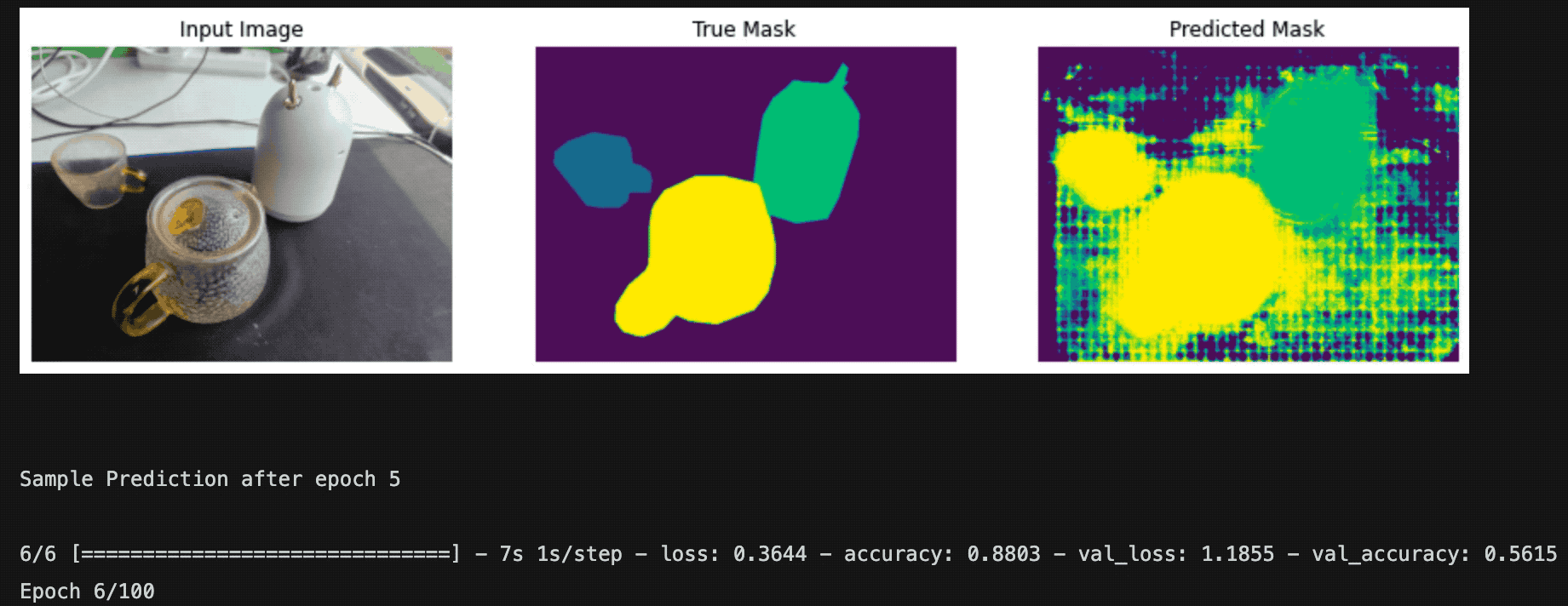## Dense-Gated U-Net (DGNet): Brain Lesion Segmentation (Biomedical Image Segmentation)## ASHISH RANA

Mentoring @Manning liveProject: RL for Self-Driving Vehicles

## Posterizing Images using KMeans Clustering (color compression)## Gradient Editing On The Fly in Deep Neural Networks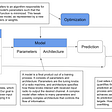## Transfer learning and active learning to find images of a small class in an unlabeled dataset.## Why initialize weights randomly(numpy.random.randn()) in Hidden Layered Neural Networks ?